Get instant live expert help with Excel or Google Sheets“My Excelchat expert helped me in less than 20 minutes, saving me what would have been 5 hours of work!”

#### Post your problem and you’ll get expert help in seconds.

Your message must be at least 40 characters
Our professional experts are available now. Your privacy is guaranteed.

# Get year from dateRead time: 15 minutes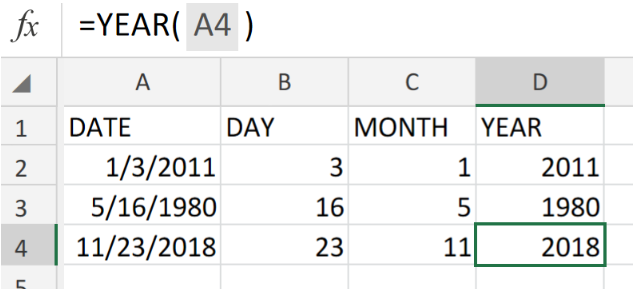Figure 1. Get Year From Date in Excel.

If we have a date list which we want to extract the year from, we are going to make use of the Excel YEAR Function.

## Generic Formula

`=YEAR(date)`

The YEAR Function operates by taking one criteria, the date from which you want to extract the year, and extracts the year from it.

## How to use the Excel YEAR Function.

We are going to demonstrate the usage of the YEAR Function in 3 simple steps.

1. Label the columns of our worksheet and assign a blank cell for Excel to determine the year number.

See example illustrated below;;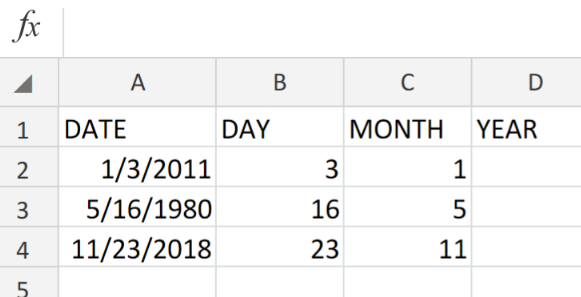Figure 2. Get Year From Date in Excel.

1. Enter the formula for the YEAR Function into the formula bar for cell D2, to generate the year number from our date list in cell A2.

The modified YEAR formula is as follows;

`=YEAR(A2)`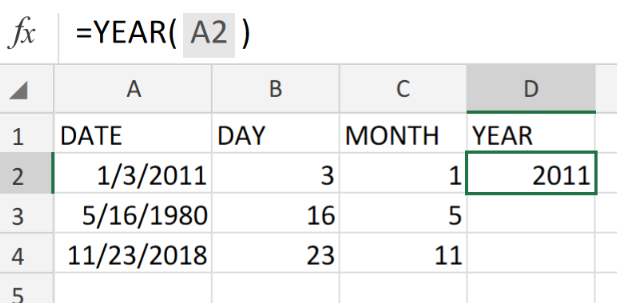Figure 3. YEAR Function in Excel.

1. Modify, copy and paste the formula in cell D2 above into the other cells in the YEAR column to get the desired results.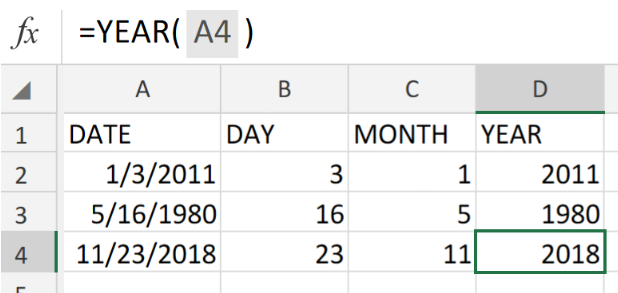Figure 4. YEAR Function in Excel.

## Note

• The dates entered in our date list have to appear in a form recognized as valid by Excel.
• Making use of dates in the form of text will only cause unpredictable results due to different regional date settings on our computers.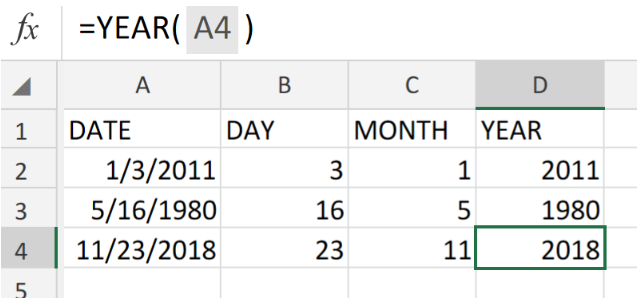Figure 5. Final Result

## Instant Connection to an Expert through our Excelchat Service:

Our live Excelchat Service is here for you. We have Excel Experts available 24/7 to answer any Excel questions you may have. Guaranteed connection within 30 seconds and a customized solution for you within 20 minutes.

### Did this post not answer your question? Get a solution from connecting with the expert.Another blog reader asked this question today on Excelchat:
Solution examplesI need a formula that looks up if the date (which looks like "8/1/2018 8:05:36 AM") in Column "C" contains year 2018. And then populates "2018" in Column D, if the date does not contain "2018" then enter False in Column D.
Solved by F. H. in 20 minscan you teach me the steps to create a nested if function, and to nest an AND function inside of an IF function?
Solved by A. Q. in 22 minsa date formula that results in showing the date as 29/12/00 (date format as dd/mm/yy) - use column BA to calculate 364 days (same date format) in column BS, if BA blank, then calculate 364 days using column AX. Results for column BA are correct. However, if column BA is empty, then it should calculate using column AX, which has data, but the result is always 29/12/00, regardless of the date in column AX. I have used this formula with success in another workbook, but this file wont work! Formula: =IF(AND(LEN(\$BA74)=0,LEN(\$AX74)=0),"",IF(LEN(\$BA74)=0,DATE(YEAR(\$AX74),MONTH(\$AX74),DAY(\$AX74)+364),DATE(YEAR(\$BA74),MONTH(\$BA74),DAY(\$BA74)+364)))
Solved by A. L. in 60 minsneed help in this =IF(C2="","",IF(ISBLANK(IF(C2="yes",HLOOKUP(VALUE(MONTH(A2) & "/" & DAY(A2) & "/" & YEAR(A2)),Sheet2!\$B\$1:\$AA\$1000,2,FALSE),IF(C2="no",HLOOKUP(VALUE(MONTH(A2) & "/" & DAY(A2) & "/" & YEAR(A2)),Sheet2!\$B\$1:\$AA\$1000,3,FALSE),""))),"NOT YET",IF(C2="yes",HLOOKUP(VALUE(MONTH(A2) & "/" & DAY(A2) & "/" & YEAR(A2)),Sheet2!\$B\$1:\$AA\$1000,2,FALSE),IF(C2="no",HLOOKUP(VALUE(MONTH(A2) & "/" & DAY(A2) & "/" & YEAR(A2)),Sheet2!\$B\$1:\$AA\$1000,3,FALSE),""))))
Solved by V. Q. in 11 minsneed help =IF(C2="","",IF(ISBLANK(IF(C2="yes",HLOOKUP(VALUE(MONTH(A2) & "/" & DAY(A2) & "/" & YEAR(A2)),Sheet2!\$B\$1:\$AA\$1000,2,FALSE),IF(C2="no",HLOOKUP(VALUE(MONTH(A2) & "/" & DAY(A2) & "/" & YEAR(A2)),Sheet2!\$B\$1:\$AA\$1000,3,FALSE),""))),"NOT YET",IF(C2="yes",HLOOKUP(VALUE(MONTH(A2) & "/" & DAY(A2) & "/" & YEAR(A2)),Sheet2!\$B\$1:\$AA\$1000,2,FALSE),IF(C2="no",HLOOKUP(VALUE(MONTH(A2) & "/" & DAY(A2) & "/" & YEAR(A2)),Sheet2!\$B\$1:\$AA\$1000,3,FALSE),""))))
Solved by G. S. in 9 mins## Subscribe to Excelchat.coAnother blog reader asked this question today on Excelchat: